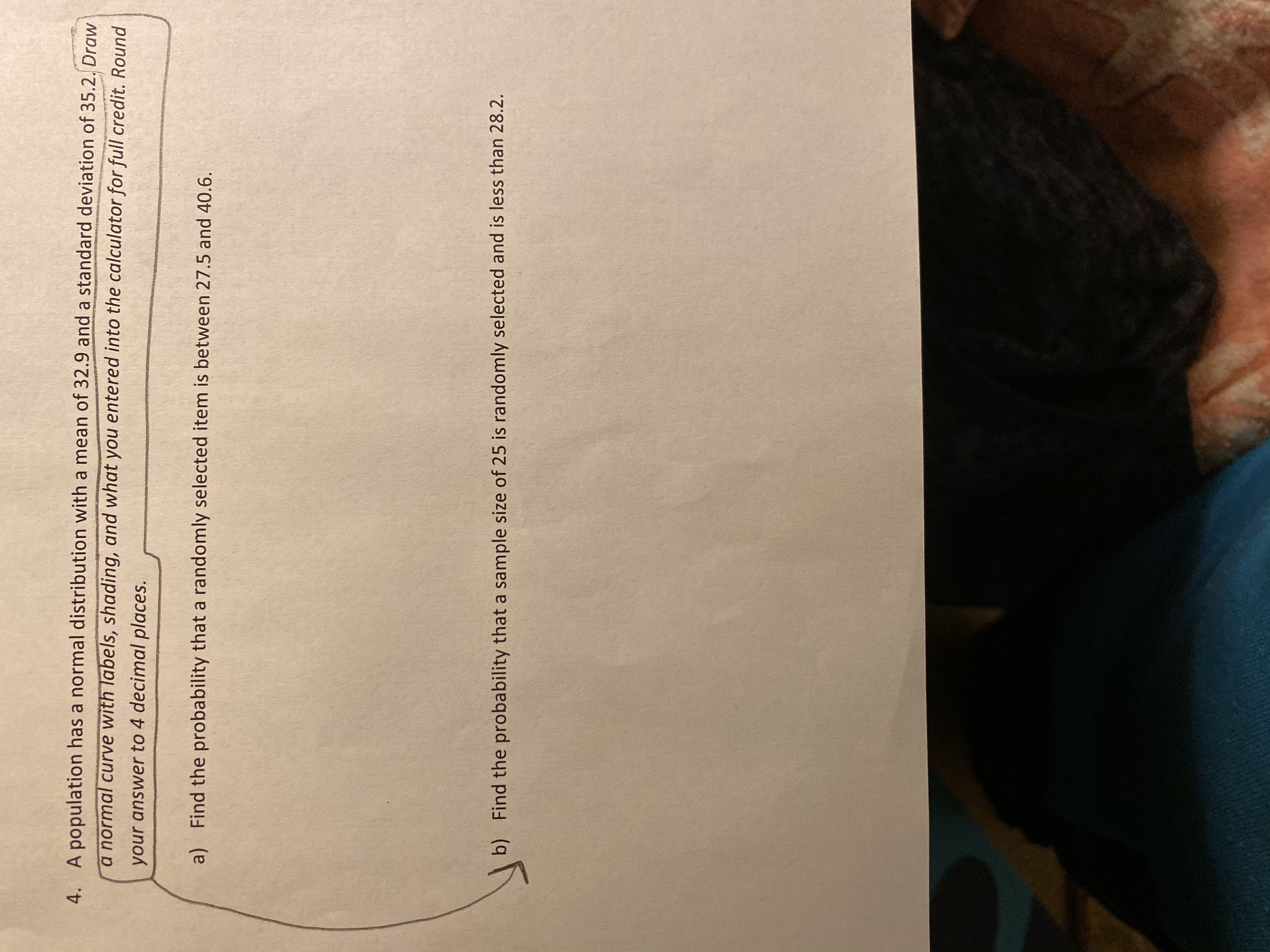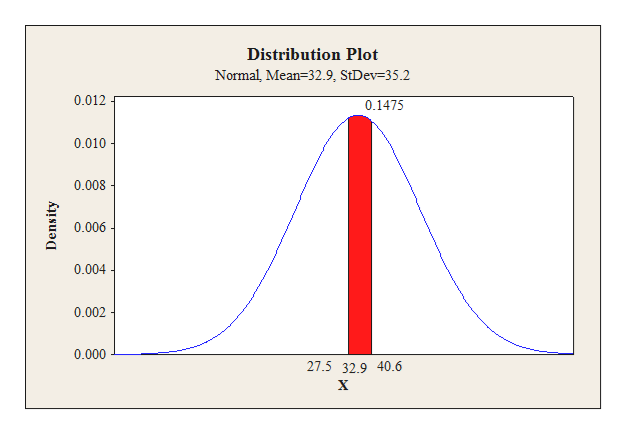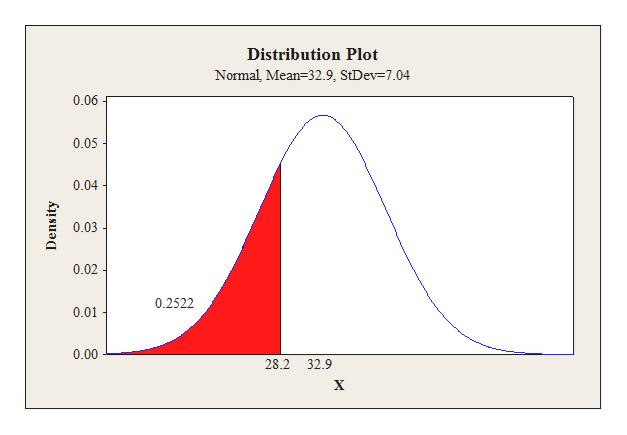# A population has a normal distribution with a mean of 32.9 and a standard deviation of 35.2. Draw4.a normal curve with labels, shading, and what you entered into the calculator for full credit. Roundyour answer to 4 decimal places.Find the probability that a randomly selected item is between 27.5 and 40.6a)Find the probability that a sample size of 25 is randomly selected and is less than 28.2.b)

Questionhelp_outlineImage TranscriptioncloseA population has a normal distribution with a mean of 32.9 and a standard deviation of 35.2. Draw 4. a normal curve with labels, shading, and what you entered into the calculator for full credit. Round your answer to 4 decimal places. Find the probability that a randomly selected item is between 27.5 and 40.6 a) Find the probability that a sample size of 25 is randomly selected and is less than 28.2. b) fullscreen
check_circle

Step 1

a)

The probability that a randomly selected item is between 27.5 and 40.6 is obtained below:

The value of mean μ = 32.9 and standard deviation is 35.2

The following normal curve corresponds to P (27.5 ≤ X ≤ 40.6) is obtained below:

The P (27.5 ≤ X ≤ 40.6) is developed using MINITAB:

• Choose Graph > Probability Distribution Plot choose View Probability > OK.
• From Distribution, choose ‘Normal’ distribution.
• In Mean enter the value as 32.9.
• In Standard deviation enter the value as 35.2.
• Choose X Value and Middle for the region of the curve to shade.
• Enter the X value 1 as 27.5.
• Enter the X value 2 as 40.6.
• Click OK.

Follow the above instructions to obtain the MINITAB output.help_outlineImage TranscriptioncloseDistribution Plot Normal, Mean-32.9, StDev-35.2 0.012 0.1475 0.010 0.008 0.006 0.004 0.002 0.000 27.5 32.9 40.6 Density fullscreen
Step 2

From the MINITAB output the value of P (27.5 ≤ X ≤ 40.6) is 0.1475.

(b)

The probability that a sample size 25 is randomly selected and is less than 28.2 is obtained below:

By central limit theorem, the sample size is 25 with mean μ = 32.9 and standard deviation, 35.2 /sqrt (25) = 7.04.

The following normal curve corresponds to P (X < 28.2) is obtained below:

The P (X < 28.2) is developed using MINITAB:

• Choose Graph > Probability Distribution Plot choose View Probability > OK.
• From Distribution, choose ‘Normal’ distribution.
• In Mean enter the va...help_outlineImage TranscriptioncloseDistribution Plot Normal, Mean 32.9, StDev 7.04 0.06 0.05 0.04 0.03 0.02 0.2522 0.01 0.00 28.2 32.9 Density fullscreen

### Want to see the full answer?

See Solution

#### Want to see this answer and more?

Solutions are written by subject experts who are available 24/7. Questions are typically answered within 1 hour.*

See Solution
*Response times may vary by subject and question.
Tagged in

### Other